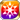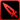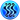# Wings UnbrokenThe Desert Wings... I thought I knew the capabilities of the Sand Hawks' rival guild well enough, but it appears that I was mistaken. "It's not the assassin's blade that disturbs my nap—just your grumbling." Not even bothering to turn his head, the guild's captain, sprawled on the floor, makes it clear that he has left everything in the hands of his self-stylized butler, Ankh. This was not out of laziness, however, but rather trust. As his gun spear intercepts the assassin Najam's blade, showering sparks, Ankh's cold stare seems to proclaim that anywhere his feet are planted shall be treated as the territory of the Desert Wings. (Carla's Recollection)

### Wings Unbroken

Raises HP by 40%/Pierce ATK Power by 30%/PATK by 30%/Strong vs Gluttony by 30%/Strong vs Envy by 30% for the Memento group "Desert Dwellers"

Target Buff (SelfSide) (CardLsSkill)
Stats
TypeMinMax
HP+40%+40%
Pierce ATK Power+30+30
PATK+30%+30%
Strong vs <暴食>+30+30
Strong vs <嫉妬>+30+30

## Stats

#### Simple stats

TypeLvl 1Lvl 30Lvl 40
AGI+2+5+7

LevelAGI
1+2
2+2
3+2
4+2
5+2
6+2
7+2
8+2
9+3
10+3
LevelAGI
11+3
12+3
13+3
14+3
15+3
16+3
17+4
18+4
19+4
20+4
LevelAGI
21+4
22+4
23+4
24+4
25+5
26+5
27+5
28+5
29+5
30+5
LevelAGI
31+5
32+5
33+6
34+6
35+6
36+6
37+6
38+6
39+6
40+7

## Group skills

### Desert Zone's Defense

#### Simple stats

TypeLvl 1Lvl 30Lvl 40
PDEF+15+41+50

LevelPDEF
1+15
2+15
3+16
4+17
5+18
6+19
7+20
8+21
9+22
10+23
LevelPDEF
11+23
12+24
13+25
14+26
15+27
16+28
17+29
18+30
19+31
20+32
LevelPDEF
21+32
22+33
23+34
24+35
25+36
26+37
27+38
28+39
29+40
30+41
LevelPDEF
31+41
32+42
33+43
34+44
35+45
36+46
37+47
38+48
39+49
40+50

#### Limit Break

TypeLB1LB2LB3LB4LB5
PDEF+6+12+18+24+30

### Desert Zone's Defense

#### Simple stats

TypeLvl 1Lvl 30Lvl 40
PDEF+15+41+50

LevelPDEF
1+15
2+15
3+16
4+17
5+18
6+19
7+20
8+21
9+22
10+23
LevelPDEF
11+23
12+24
13+25
14+26
15+27
16+28
17+29
18+30
19+31
20+32
LevelPDEF
21+32
22+33
23+34
24+35
25+36
26+37
27+38
28+39
29+40
30+41
LevelPDEF
31+41
32+42
33+43
34+44
35+45
36+46
37+47
38+48
39+49
40+50

#### Limit Break

TypeLB1LB2LB3LB4LB5
PDEF+6+12+18+24+30

### United Front

#### Simple stats

TypeLvl 1Lvl 30Lvl 40
PATK+20+49+60
Paralyze Res+10+24+30

#### Detailed stats

LevelPATKParalyze Res
1+20+10
2+21+10
3+22+11
4+23+11
5+24+12
6+25+12
7+26+13
8+27+13
9+28+14
10+29+14
LevelPATKParalyze Res
11+30+15
12+31+15
13+32+16
14+33+16
15+34+17
16+35+17
17+36+18
18+37+18
19+38+19
20+39+19
LevelPATKParalyze Res
21+40+20
22+41+20
23+42+21
24+43+21
25+44+22
26+45+22
27+46+23
28+47+23
29+48+24
30+49+24
LevelPATKParalyze Res
31+50+25
32+51+25
33+52+26
34+53+26
35+54+27
36+55+27
37+56+28
38+57+28
39+58+29
40+60+30

#### Limit Break

TypeLB1LB2LB3LB4LB5
PATK+7+14+21+28+36
Paralyze Res+4+7+11+14+18

### Gun Spear Bridge

#### Simple stats

TypeLvl 1Lvl 30Lvl 40
Strong vs Fire+5+12+15
Water+3+6+8

#### Detailed stats

LevelStrong vs FireWater
1+5+3
2+5+3
3+5+3
4+5+3
5+6+3
6+6+3
7+6+3
8+6+3
9+7+4
10+7+4
LevelStrong vs FireWater
11+7+4
12+7+4
13+8+4
14+8+4
15+8+4
16+8+4
17+9+5
18+9+5
19+9+5
20+9+5
LevelStrong vs FireWater
21+10+5
22+10+5
23+10+5
24+10+5
25+11+6
26+11+6
27+11+6
28+11+6
29+12+6
30+12+6
LevelStrong vs FireWater
31+12+6
32+12+6
33+13+7
34+13+7
35+13+7
36+13+7
37+14+7
38+14+7
39+14+7
40+15+8

#### Limit Break

TypeLB1LB2LB3LB4LB5
Strong vs Fire+2+3+5+7+9
Water+1+2+3+4+5

#### Max Limit Break

TypeLB5
Jewels Spent Rate-15

## Vision abilities

### Limit Reached Bonus

#### Ability base

• Slot:Support

Revealed after Limit Reached. Raises AGI after appearing on map.

#### Ability max

• Slot:Action

Raises AGI after appearing on map.

### Prideful Wings

#### Ability base

• Slot:Action
• DMG Type:Physical
• ATK Type:Pierce
• Element:Water

Water Pierce ATK on enemy unit & chance of critical & moves target 3sq back & disables all Reactions to this attack [Range: 1, Height Range: 2]

Skill Effect
TypeMinMax
Attack133%153%
• Charges: 2
• Jewel Cost: 45
• Height: 2
• Select Range: Cross
• Range: 0-1

#### Ability max

• Slot:Action
• DMG Type:Physical
• ATK Type:Pierce
• Element:Water

Water Pierce ATK on enemy unit & chance of critical & moves target 3sq back & disables all Reactions to this attack [Range: 1, Height Range: 2]

Skill Effect
TypeMinMax
Attack245%245%
• Charges: 2
• Jewel Cost: 45
• Height: 2
• Select Range: Cross
• Range: 0-1

#### Unit and job restriction

[[Category:Strong vs <暴食>]][[Category:Strong vs <嫉妬>]]